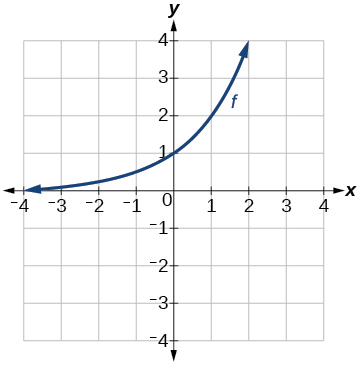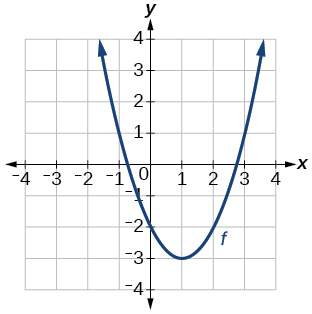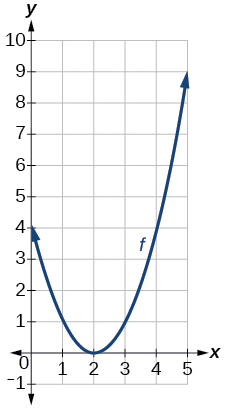# 1.5E: Exercises

This page is a draft and is under active development.

$$\newcommand{\vecs}{\overset { \rightharpoonup} {\mathbf{#1}} }$$ $$\newcommand{\vecd}{\overset{-\!-\!\rightharpoonup}{\vphantom{a}\smash {#1}}}$$$$\newcommand{\id}{\mathrm{id}}$$ $$\newcommand{\Span}{\mathrm{span}}$$ $$\newcommand{\kernel}{\mathrm{null}\,}$$ $$\newcommand{\range}{\mathrm{range}\,}$$ $$\newcommand{\RealPart}{\mathrm{Re}}$$ $$\newcommand{\ImaginaryPart}{\mathrm{Im}}$$ $$\newcommand{\Argument}{\mathrm{Arg}}$$ $$\newcommand{\norm}{\| #1 \|}$$ $$\newcommand{\inner}{\langle #1, #2 \rangle}$$ $$\newcommand{\Span}{\mathrm{span}}$$ $$\newcommand{\id}{\mathrm{id}}$$ $$\newcommand{\Span}{\mathrm{span}}$$ $$\newcommand{\kernel}{\mathrm{null}\,}$$ $$\newcommand{\range}{\mathrm{range}\,}$$ $$\newcommand{\RealPart}{\mathrm{Re}}$$ $$\newcommand{\ImaginaryPart}{\mathrm{Im}}$$ $$\newcommand{\Argument}{\mathrm{Arg}}$$ $$\newcommand{\norm}{\| #1 \|}$$ $$\newcommand{\inner}{\langle #1, #2 \rangle}$$ $$\newcommand{\Span}{\mathrm{span}}$$$$\newcommand{\AA}{\unicode[.8,0]{x212B}}$$

Often when given a problem, we try to model the scenario using mathematics in the form of words, tables, graphs, and equations. One method we can employ is to adapt the basic graphs of the toolkit functions to build new models for a given scenario. There are systematic ways to alter functions to construct appropriate models for the problems we are trying to solve.

Verbal

Exercise 1.5.1

When examining the formula of a function that is the result of multiple transformations, how can you tell a horizontal shift from a vertical shift?

A horizontal shift results when a constant is added to or subtracted from the input. A vertical shifts results when a constant is added to or subtracted from the output.

Exercise 1.5.2

When examining the formula of a function that is the result of multiple transformations, how can you tell a horizontal stretch from a vertical stretch?

Exercise 1.5.3

When examining the formula of a function that is the result of multiple transformations, how can you tell a horizontal compression from a vertical compression?

A horizontal compression results when a constant greater than 1 is multiplied by the input. A vertical compression results when a constant between 0 and 1 is multiplied by the output.

Exercise 1.5.4

When examining the formula of a function that is the result of multiple transformations, how can you tell a reflection with respect to the x-axis from a reflection with respect to the y-axis?

Exercise 1.5.5

How can you determine whether a function is odd or even from the formula of the function?

For a function $$f$$, substitute (−x) for (x) in $$f(x)$$. Simplify. If the resulting function is the same as the original function, $$f(−x)=f(x)$$, then the function is even. If the resulting function is the opposite of the original function, $$f(−x)=−f(x)$$, then the original function is odd. If the function is not the same or the opposite, then the function is neither odd nor even.

Algebraic

Exercise 1.5.6

Write a formula for the function obtained when the graph of $$f(x)=\sqrt{x}$$ is shifted up 1 unit and to the left 2 units.

Exercise 1.5.7

Write a formula for the function obtained when the graph of $$f(x)=|x|$$ is shifted down 3 units and to the right 1 unit.

$$g(x)=|x-1|−3$$

Exercise 1.5.8

Write a formula for the function obtained when the graph of $$f(x)=\dfrac{1}{x}$$ is shifted down 4 units and to the right 3 units.

Exercise 1.5.9

Write a formula for the function obtained when the graph of $$f(x)=\dfrac{1}{x^2}$$ is shifted up 2 units and to the left 4 units.

$$g(x)=\dfrac{1}{(x+4)^2}+2$$

For the following exercises, describe how the graph of the function is a transformation of the graph of the original function $$f$$.

Exercise 1.5.10

$$y=f(x−49)$$

Exercise 1.5.11

$$y=f(x+43)$$

The graph of $$f(x+43)$$ is a horizontal shift to the left 43 units of the graph of $$f$$.

Exercise 1.5.12

$$y=f(x+3)$$

Exercise 1.5.13

$$y=f(x−4)$$

The graph of $$f(x-4)$$ is a horizontal shift to the right 4 units of the graph of f.

Exercise 1.5.14

$$y=f(x)+5$$

Exercise 1.5.15

$$y=f(x)+8$$

The graph of $$f(x)+8$$ is a vertical shift up 8 units of the graph of $$f$$.

Exercise 1.5.16

$$y=f(x)−2$$

Exercise 1.5.17

$$y=f(x)−7$$

The graph of $$f(x)−7$$ is a vertical shift down 7 units of the graph of $$f$$.

Exercise 1.5.18

$$y=f(x−2)+3$$

Exercise 1.5.19

$$y=f(x+4)−1$$

The graph of $$f(x+4)−1$$ is a horizontal shift to the left 4 units and a vertical shift down 1 unit of the graph of $$f$$.

For the following exercises, determine the interval(s) on which the function is increasing and decreasing.

Exercise 1.5.20

$$f(x)=4(x+1)^2−5$$

Exercise 1.5.21

$$g(x)=5(x+3)^2−2$$

decreasing on $$(−\infty,−3)$$ and increasing on $$(−3,\infty)$$

Exercise 1.5.22

$$a(x)=\sqrt{−x+4}$$

Exercise 1.5.23

$$k(x)=−3\sqrt{x}−1$$

decreasing on $$(0, \infty)$$

Graphical

For the following exercises, use the graph of $$f(x)=2^x$$ shown in Figure 1.5.30 to sketch a graph of each transformation of $$f(x)$$.Figure 1.5.30: Graph of $$f(x)$$.

Exercise 1.5.24

$$h(x)=2^x−3$$

Exercise 1.5.25

$$g(x)=2^x+1$$Graph of $$g(x)$$

Exercise 1.5.26

$$w(x)=2^x−1$$

For the following exercises, sketch a graph of the function as a transformation of the graph of one of the toolkit functions.

Exercise 1.5.27

$$f(t)=(t+1)^2−3$$Graph of $$f(t)$$.

Exercise 1.5.28

$$h(x)=|x−1|+4$$

Exercise 1.5.29

$$k(x)=(x−2)^3−1$$Graph of $$k(x)$$.

Exercise 1.5.30

$$m(t)=3+\sqrt{t+2}$$

Numeric

Exercise 1.5.31

Tabular representations for the functions $$f$$, $$g$$, and $$h$$ are given below. Write $$g(x)$$ and $$h(x)$$ as transformations of $$f(x)$$.

 $$f(x)$$ $$x$$ -2 -1 0 1 2 -2 -1 -3 1 2
 $$g(x)$$ $$x$$ -1 0 1 2 3 -2 -1 -3 1 2
 $$h(x)$$ $$x$$ -2 -1 0 1 2 -1 0 -2 2 3

$$g(x)=f(x-1)$$, $$h(x)=f(x)+1$$

Exercise 1.5.32

Tabular representations for the functions $$f$$, $$g$$, and $$h$$ are given below. Write $$g(x)$$ and $$h(x)$$ as transformations of $$f(x)$$.

 $$f(x)$$ $$x$$ -2 -1 0 1 2 -1 -3 4 2 1

 $$g(x)$$ $$x$$ -3 -2 -1 0 1 -1 -3 4 2 1
 $$h(x)$$ $$x$$ -2 -1 0 1 2 -2 -4 3 1 0

For the following exercises, write an equation for each graphed function by using transformations of the graphs of one of the toolkit functions.

Exercise 1.5.33Graph of an absolute function.

$$f(x)=|x-3|−2$$

Exercise 1.5.34Graph of a parabola.

Exercise 1.5.35Graph of a square root function.

$$f(x)=\sqrt{x+3}−1$$

Exercise 1.5.36Graph of an absolute function.

Exercise 1.5.37Graph of a parabola

$$f(x)=(x-2)^2$$

Exercise 1.5.38Graph of a square root function.

Exercise 1.5.39Graph of an absolute function.

$$f(x)=|x+3|−2$$

Exercise 1.5.40Graph of a square root function.

For the following exercises, use the graphs of transformations of the square root function to find a formula for each of the functions.

Exercise 1.5.41Graph of a square root function.

$$f(x)=−\sqrt{x}$$

Exercise 1.5.42Graph of a square root function.

For the following exercises, use the graphs of the transformed toolkit functions to write a formula for each of the resulting functions.

Exercise 1.5.43Graph of a parabola.

$$f(x)=−(x+1)^2+2$$

Exercise 1.5.44Graph of a cubic function.

Exercise 1.5.45Graph of a square root function.

$$f(x)=\sqrt{−x}+1$$

Exercise 1.5.46Graph of an absolute function.

For the following exercises, determine whether the function is odd, even, or neither.

Exercise 1.5.47

$$f(x)=3x^4$$

even

Exercise 1.5.48

$$g(x)=\sqrt{x}$$

Exercise 1.5.49

$$h(x)=\dfrac{1}{x}+3x$$

odd

Exercise 1.5.50

$$f(x)=(x−2)^2$$

Exercise 1.5.51

$$g(x)=2x^4$$

even

Exercise 1.5.52

$$h(x)=2x−x^3$$

For the following exercises, describe how the graph of each function is a transformation of the graph of the original function $$f$$.

Exercise 1.5.53

$$g(x)=−f(x)$$

The graph of $$g$$ is a vertical reflection (across the x-axis) of the graph of $$f$$.

Exercise 1.5.54

$$g(x)=f(−x)$$

Exercise 1.5.55

$$g(x)=4f(x)$$

The graph of $$g$$ is a vertical stretch by a factor of 4 of the graph of $$f$$.

Exercise 1.5.56

$$g(x)=6f(x)$$

Exercise 1.5.57

$$g(x)=f(5x)$$

The graph of $$g$$ is a horizontal compression by a factor of 15 of the graph of $$f$$.

Exercise 1.5.58

$$g(x)=f(2x)$$

Exercise 1.5.59

$$g(x)=f(\frac{1}{3}x)$$

The graph of $$g$$ is a horizontal stretch by a factor of 3 of the graph of $$f$$.

Exercise 1.5.60

$$g(x)=f(\frac{1}{5}x)$$

Exercise 1.5.61

$$g(x)=3f(−x)$$

The graph of $$g$$ is a horizontal reflection across the y-axis and a vertical stretch by a factor of 3 of the graph of $$f$$.

Exercise 1.5.62

$$g(x)=−f(3x)$$

For the following exercises, write a formula for the function $$g$$ that results when the graph of a given toolkit function is transformed as described.

Exercise 1.5.63

The graph of $$f(x)=|x|$$ is reflected over the y-axis and horizontally compressed by a factor of $$\frac{1}{4}$$ .

$$g(x)=|−4x|$$

Exercise 1.5.64

The graph of $$f(x)=\sqrt{x}$$ is reflected over the x-axis and horizontally stretched by a factor of 2.

Exercise 1.5.65

The graph of $$f(x)=\dfrac{1}{x^2}$$ is vertically compressed by a factor of $$\dfrac{1}{3}$$, then shifted to the left 2 units and down 3 units.

$$g(x)=\dfrac{1}{3(x+2)^2}−3$$

Exercise 1.5.66

The graph of $$f(x)=\dfrac{1}{x}$$ is vertically stretched by a factor of 8, then shifted to the right 4 units and up 2 units.

Exercise 1.5.67

The graph of $$f(x)=x^2$$ is vertically compressed by a factor of $$\frac{1}{2}$$, then shifted to the right 5 units and up 1 unit.

$$g(x)=\dfrac{1}{2}(x-5)^2+1$$

Exercise 1.5.68

The graph of $$f(x)=x^2$$ is horizontally stretched by a factor of 3, then shifted to the left 4 units and down 3 units.

For the following exercises, describe how the formula is a transformation of a toolkit function. Then sketch a graph of the transformation.

Exercise 1.5.69

$$g(x)=4(x+1)^2−5$$

The graph of the function $$f(x)=x^2$$ is shifted to the left 1 unit, stretched vertically by a factor of 4, and shifted down 5 units.Graph of a parabola.

Exercise 1.5.70

$$g(x)=5(x+3)^2−2$$

Exercise 1.5.71

$$h(x)=−2|x−4|+3$$

The graph of $$f(x)=|x|$$ is stretched vertically by a factor of 2, shifted horizontally 4 units to the right, reflected across the horizontal axis, and then shifted vertically 3 units up.Graph of an absolute value function.

Exercise 1.5.72

$$k(x)=−3\sqrt{x}−1$$

Exercise 1.5.73

$$m(x)=\dfrac{1}{2}x^3$$

The graph of the function $$f(x)=x^3$$ is compressed vertically by a factor of $$\dfrac{1}{2}$$.Graph of a cubic function.

Exercise 1.5.74

$$n(x)=\dfrac{1}{3}|x−2|$$

Exercise 1.5.75

$$p(x)=(\dfrac{1}{3}x)^3−3$$

The graph of the function is stretched horizontally by a factor of 3 and then shifted vertically downward by 3 units.Graph of a cubic function.

Exercise 1.5.76

$$q(x)=(\dfrac{1}{4}x)^3+1$$

Exercise 1.5.77

$$a(x)=\sqrt{−x+4}$$

The graph needs work. Wait for the revised edition!

For the following exercises, use the graph in Figure 1.5.30 to sketch the given transformations.Figure 1.5.30: Graph of a polynomial.

Exercise 1.5.78

$$g(x)=f(x)−2$$

Exercise 1.5.79

$$g(x)=−f(x)$$Graph of a polynomial.

Exercise 1.5.80

$$g(x)=f(x+1)$$

Exercise 1.5.81

$$g(x)=f(x−2)$$